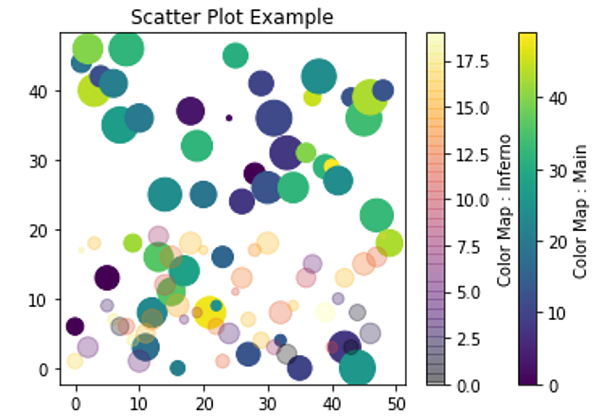# Python | Overlapping Scatter Plot Example

In this tutorial, we are going to learn how to do overlap scatter plotting in python using matplotlib?
Submitted by Anuj Singh, on August 10, 2020

It is one of the most used scatter plot techniques when there is multiple data comparison. We generally change the opaque intensity and color map for better visualization.

The following example shows the implementation of overlap scatters plotting with two different cmaps.## Python code for overlapping scatter plot example

```import numpy as np
import matplotlib.pyplot as plt

xy1 = [1,2,3,4,5,6]
xy2 = [4,8,7,15,9.6, 4.5]
xy3 = [4.9,8.9,17,18,19.6, 8.9]

x = np.arange(50)
y = np.random.randint(0,50,50)
ss = np.random.randint(0,50,50)
c = np.random.randint(0,50,50)

plt.figure()
plt.scatter(x,y, s=ss*10, c=c)
plt.axvline(17, ymin=7, ymax=37, linewidth=4.0)
plt.title('Scatter Plot Example')
plt.colorbar(label='Color Map : Main')

x = np.arange(50)
y = np.random.randint(0,20,50)
ss = np.random.randint(0,20,50)
c = np.random.randint(0,20,50)

plt.scatter(x,y, s=ss*10, c=c, cmap='inferno', alpha=0.3)
plt.axvline(17, ymin=7, ymax=37, linewidth=4.0)
plt.title('Scatter Plot Example')
plt.colorbar(label='Color Map : Inferno')
plt.show()
```

Output:

```Output is as Figure
```

Languages: » C » C++ » C++ STL » Java » Data Structure » C#.Net » Android » Kotlin » SQL
Web Technologies: » PHP » Python » JavaScript » CSS » Ajax » Node.js » Web programming/HTML
Solved programs: » C » C++ » DS » Java » C#
Aptitude que. & ans.: » C » C++ » Java » DBMS
Interview que. & ans.: » C » Embedded C » Java » SEO » HR
CS Subjects: » CS Basics » O.S. » Networks » DBMS » Embedded Systems » Cloud Computing
» Machine learning » CS Organizations » Linux » DOS
More: » Articles » Puzzles » News/Updates